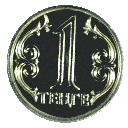# On MeasurementI read this the other day, as part of a discussion about monies; in particular the fact that at one point in history not all currencies were divided into decimals, as the US Dollar is (ten pennies = one dime, ten dimes = one dollar). I believe it’s originally from Terry Pratchett’s book Good Omens:

“Two farthings = One Ha’penny. Two ha’pennies = One Penny. Three pennies = A Thrupenny Bit. Two Thrupences = A Sixpence. Two Sixpences = One Shilling, or Bob. Two Bob = A Florin. One Florin and one Sixpence = Half a Crown. Four Half Crowns = Ten Bob Note. Two Ten Bob Notes = One Pound (or 240 pennies). One Pound and One Shilling = One Guinea.

In the UK they resisted decimal currency for a long time because they thought that “100 pennies to 1 pound” was too complicated”

In comparison, I offer this:

Twelve inches = one foot. Three feet = One Yard. 1,760 yards (or 5,280 feet, or 63,360 inches) = One Mile.

Is “100 centimeters to 1 meter, 1000 meters to 1 kilometer” really too complicated for us in the U.S.?